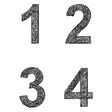# Generators and Iterators in Julia and Python

`julia> g = (x*x for x in 1:4)Base.Generator{UnitRange{Int64},getfield(Main, Symbol("##27#28"))}(getfield(Main, Symbol("##27#28"))(), 1:4)`
`python> g = (x*x for x in range(1,5))python> g<generator object <genexpr> at 0x10bdeef48>`

## Python Iterators

`class Point:    def __init__(self, x, y, z):        self.x = x        self.y = y        self.z = z`
`python> p = Point(3, 8, 2)`
`def __iter__(self):        return PointIterator(self)`
`def __repr__(self):        return f"Point({self.x}, {self.y}, {self.z})"`
`class PointIterator:    def __init__(self, point):        self.point = point        self.coords = ['z', 'y', 'x']    def __next__(self):        if self.coords:            return getattr(self.point, self.coords.pop())        else:            raise StopIteration`
`python> p = Point(2, 3, 8)python> for a in p:    print(a)238python> it = iter(p)python> next(it)2python> next(it)3python> next(it)8`

## Julia Iterators

`struct Point		x::Int		y::Int		z::Intend`
`for i in iter   # or  "for i = iter"    # bodyend`
`next = iterate(iter)while next !== nothing    (i, state) = next    # body    next = iterate(iter, state)end`
`iterate(p::Point) = p.x, [:y, :z]function iterate(p::Point, coords)   if isempty(coords)       nothing   else       getfield(p, coords), coords[2:end]   endend`
`python> it = enumerate(["one", "two"])python> list(it)[(0, 'one'), (1, 'two')]python> list(it)[]`
`julia> it = enumerate(["one", "two"])Base.Iterators.Enumerate{Array{String,1}}(["one", "two"])julia> collect(it)2-element Array{Tuple{Int64,String},1}: (1, "one") (2, "two")julia> collect(it)2-element Array{Tuple{Int64,String},1}: (1, "one") (2, "two")`

## Using Python Generators to Implement Iterators

`class Point:    def __init__(self, x, y, z):        self.x = x        self.y = y        self.z = z        def __repr__(self):        return f"({self.x}, {self.y}, {self.z})"            def __iter__(self):        def gen():            yield self.x            yield self.y            yield self.z        return gen()`
`def generator():    for i in range(0, 6):        yield chr(ord('A') + i)`
`python> g = generator()python> list(g)['A', 'B', 'C', 'D', 'E', 'F']python> g = generator()python> next(g)'A'python> next(g)'B'python> next(g)'C'`

## Python Style Generator in Julia

`function generator()    Channel() do channel        for i in 0:5            put!(channel, Char('A' + i))        end    endend`
`julia> g = generator()Channel{Any}(sz_max:0,sz_curr:1)`
`julia> collect(g)6-element Array{Any,1}: 'A' 'B' 'C' 'D' 'E' 'F'`
`julia> g = generator()julia> [g...]6-element Array{Char,1}: 'A' 'B' 'C' 'D' 'E' 'F'`
`julia> g = generator()julia> string(g...)"ABCDEF"`

`function generator()   channel = Channel(2)   function make_letters()       for i in 0:5           put!(channel, Char('A' + i))       end   end   task = Task(make_letters)   schedule(task)   channelend`
`put!(channel, Char('A' + i))`
`task = Task(make_letters)`
`schedule(task)`
`julia> channel = generator()julia> take!(ch)'A'`
`function generator()   channel = Channel(2)   @async begin       for i in 0:5           put!(channel, Char('A' + i))       end   end   channelend`
`function generator()   function make_letters(channel::Channel)       for i in 0:5           put!(channel, Char('A' + i))       end   end   Channel(make_letters)end`
`foobar(x -> stuff(x), y, z)foobar(y, z) do x stuff(x)end`

--

--

--

## More from Erik Engheim

Geek dad, living in Oslo, Norway with passion for UX, Julia programming, science, teaching, reading and writing.

Love podcasts or audiobooks? Learn on the go with our new app.

## WSO2 Product Installers for Popular Operating Systems## Using a GPU Boosts TiDB Analytics Performance by 10 to 150 Times## How to Use Network Load Balancer to Connect to OCI Instances## Gradient Toolbar in the Android App## Best POS Software for Super Shops, Grocery Stores, Restaurants, Pharmacies, Mudi Dokans, Kirana…## Software Patents -“That’s Where All The Action Is”## Guidelines to implement the MVVM pattern in Android## Erik Engheim

Geek dad, living in Oslo, Norway with passion for UX, Julia programming, science, teaching, reading and writing.

## Everything you need to know about Indexing in Julia## Progressbars (tqdm and likes) in Julia## Hacking the Python syntax: Ternary operator## Introduction to Makefiles